JEE  >  Test: MCQs (One or More Correct Option): Solutions | JEE Advanced

# Test: MCQs (One or More Correct Option): Solutions | JEE Advanced

Test Description

## 17 Questions MCQ Test Chemistry 35 Years JEE Main & Advanced Past year Papers | Test: MCQs (One or More Correct Option): Solutions | JEE Advanced

Test: MCQs (One or More Correct Option): Solutions | JEE Advanced for JEE 2022 is part of Chemistry 35 Years JEE Main & Advanced Past year Papers preparation. The Test: MCQs (One or More Correct Option): Solutions | JEE Advanced questions and answers have been prepared according to the JEE exam syllabus.The Test: MCQs (One or More Correct Option): Solutions | JEE Advanced MCQs are made for JEE 2022 Exam. Find important definitions, questions, notes, meanings, examples, exercises, MCQs and online tests for Test: MCQs (One or More Correct Option): Solutions | JEE Advanced below.
 1 Crore+ students have signed up on EduRev. Have you?
Test: MCQs (One or More Correct Option): Solutions | JEE Advanced - Question 1

### An azeotropic solution of two liquids has boiling point lowerthan either of them when it      (1981 - 1 Mark)

Detailed Solution for Test: MCQs (One or More Correct Option): Solutions | JEE Advanced - Question 1

Lower the B. pt., higher will be the V.P. The V.P. of the mixture is greater than either of the two liquids.
[NOTE : : In case of positive deviation from Roult's law the partial vapour pressure of each liquid and total vapour pressure of solution will be greater as compared to initial solution]

Test: MCQs (One or More Correct Option): Solutions | JEE Advanced - Question 2

### For a dilute solution, Raoult’s law states that :(1985 - 1 Mark)

Detailed Solution for Test: MCQs (One or More Correct Option): Solutions | JEE Advanced - Question 2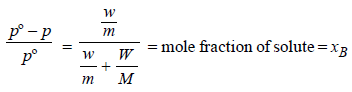[Mathematical statement of Raoult's law]

Test: MCQs (One or More Correct Option): Solutions | JEE Advanced - Question 3

### When mercuric iodide is added to the aqueous solution ofpotassium iodide then (1987 - 1 Mark)

Detailed Solution for Test: MCQs (One or More Correct Option): Solutions | JEE Advanced - Question 3

Added HgI2 forms a complex with KI in the solution as follows
2KI + HgI2 → K2[HgI4]
As a result, number of particles decreases and so ΔTf increases.
[NOTE : :
Depression in freezing point is a colligative property]

Test: MCQs (One or More Correct Option): Solutions | JEE Advanced - Question 4

Which of the following 0.1 M aqueous solutions will havethe lowest freezing point? (1989 - 1 Mark)

Detailed Solution for Test: MCQs (One or More Correct Option): Solutions | JEE Advanced - Question 4

NOTE : The salt producing highest number of ions will have lowest freezing point.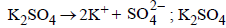gives highest number of particles (2 + 1 = 3).
Glucose, being non-electrolyte gives minimum no. of particles and hence minimum ΔTf or maximum F. pt.

Test: MCQs (One or More Correct Option): Solutions | JEE Advanced - Question 5

The freezing point of equimolal aqueous solutions will behighest for : (1990 - 1 Mark)

Detailed Solution for Test: MCQs (One or More Correct Option): Solutions | JEE Advanced - Question 5

TIPS/Formulae : The salt that ionises to least extent will have highest freezing point. [ i.e., minimum Δ Tf ]
Glucose, being non electrolyte, gives minimum no. of particles and hence minimum DTf or maximum F. pt

Test: MCQs (One or More Correct Option): Solutions | JEE Advanced - Question 6

0.2 molal acid HX is 20% ionised in solution. Kf = 1.86 Kmolality–1. The freezing point of the solution is : (1995S)

Detailed Solution for Test: MCQs (One or More Correct Option): Solutions | JEE Advanced - Question 6

Depression in freezing point,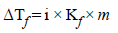Van’t Hoff factor,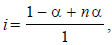where n = no. of ions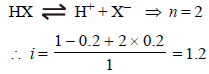[ For 20 % ionisation, α = 20/100= 0.2]
∴ΔTf = 1.2 × 1.86 × 0.2 = 0.45 [ ∵ m = 0.2]
Hence freezing point of solution is 0 – 0.45 = –0.45
[∵ F.P of water = 0.0 C]

Test: MCQs (One or More Correct Option): Solutions | JEE Advanced - Question 7

The molecular weight of benzoic acid in benzene asdetermined by depression in freezing point methodcorresponds to : (1996 - 1 Mark)

Detailed Solution for Test: MCQs (One or More Correct Option): Solutions | JEE Advanced - Question 7

Benzoic acid exists as dimer in benzene.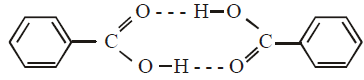[Normal molecular mass = 122 amu
observed molecular mass = 244 amu, in case of complete association]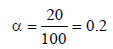Test: MCQs (One or More Correct Option): Solutions | JEE Advanced - Question 8

During depression of freezing point in a solution thefollowing are in equililbrium (2003S)

Detailed Solution for Test: MCQs (One or More Correct Option): Solutions | JEE Advanced - Question 8

NOTE : At the freezing point liquid and solid remain in equilibrium. If a solution of a non-volatile solute is cooled to a temperature below the freezing point of solution, some of liquid solvent will separate as a solid solvent and thus the concentration of solution will
increase.

Test: MCQs (One or More Correct Option): Solutions | JEE Advanced - Question 9

The elevation in boiling point of a solution of 13.44 g ofCuCl2 in 1 kg of water using the following information will be(Molecular weight of CuCl2 = 134.4 and Kb = 0.52 K molal-1)       (2005S)

Detailed Solution for Test: MCQs (One or More Correct Option): Solutions | JEE Advanced - Question 9

TIPS/Formulae :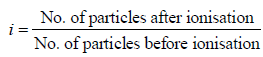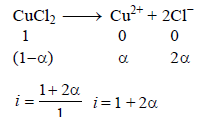Assuming 100% ionization So, i = 1 + 2 = 3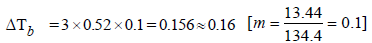Test: MCQs (One or More Correct Option): Solutions | JEE Advanced - Question 10

When 20 g of naphthoic acid (C11H8O2) is dissolved in 50g of benzene (Kf = 1.72 K kg mol–1), a freezing pointdepression of 2K is observed. The Van't Hoff factor (i) is            (2007)

Detailed Solution for Test: MCQs (One or More Correct Option): Solutions | JEE Advanced - Question 10

Molecular weight of naphthoic acid
C11H8O2 = 172 gmol–1.
The theoretical value of depression in freezing point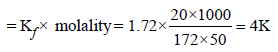Van't Hoff factor,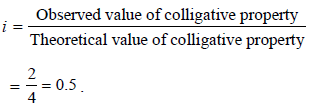Test: MCQs (One or More Correct Option): Solutions | JEE Advanced - Question 11

The Henry’s law constant for the solubility of N2 gas inwater at 298 K is 1.0 × 105 atm. The mole fraction of N2 in airis 0.8. The number of moles of N2 from air dissolved in10 moles of water at 298 K and 5 atm pressure is (2009)

Detailed Solution for Test: MCQs (One or More Correct Option): Solutions | JEE Advanced - Question 11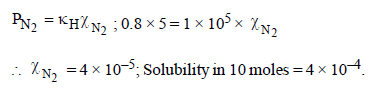Test: MCQs (One or More Correct Option): Solutions | JEE Advanced - Question 12

Dissolving 120 g of urea (mol. wt. 60) in 1000 g of water gavea solution of density 1.15 g/mL. The molarity of the solutionis (2011)

Detailed Solution for Test: MCQs (One or More Correct Option): Solutions | JEE Advanced - Question 12

Number of moles of urea = 120/60 = 2

Total mass of solution = 1000 + 120 = 1120 g
Total volume of solution (in L) = Mass/density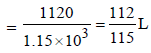Molarity of the solution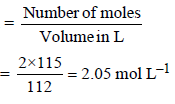Test: MCQs (One or More Correct Option): Solutions | JEE Advanced - Question 13

The freezing point (in °C) of a solution containing 0.1 gof K3[Fe(CN)6] (Mol. wt. 329) in 100 g of water (Kf = 1.86 Kkg mol–1) is (2011)

Detailed Solution for Test: MCQs (One or More Correct Option): Solutions | JEE Advanced - Question 13

ΔTf = i × Kf × m
Where m = Molality of the solution
(i.e. number of moles of solute per 1000 g of the solvent)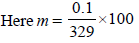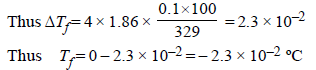Test: MCQs (One or More Correct Option): Solutions | JEE Advanced - Question 14

For a dilute solution containing 2.5 g of a non-volatile nonelectrolytesolute in 100 g of water, the elevationin boiling point at 1 atm pressure is 2°C. Assumingconcentration of solute is much lower than the concentrationof solvent, the vapour pressure (mm of Hg) of the solutionis (take Kb = 0.76 K kg mol–1) (2012)

Detailed Solution for Test: MCQs (One or More Correct Option): Solutions | JEE Advanced - Question 14

From Raoult law relation,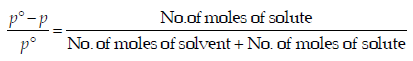When the concentration of solute is much lower than the concentration of solvent,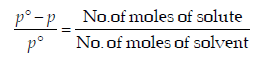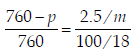.... (i)

From elevation in boiling point, ΔTb = Kb × m
2 = 0.76 × m
m = 2/0.76              ...(ii)
From (i) and (ii), p = 724 mm

*Multiple options can be correct
Test: MCQs (One or More Correct Option): Solutions | JEE Advanced - Question 15

In the depression of freezing point experiment, it is foundthat the (1999 - 3 Marks)

Detailed Solution for Test: MCQs (One or More Correct Option): Solutions | JEE Advanced - Question 15

(a, d) The freezing point of a solvent depresses as a nonvolatile solute is added to a solvent. According to Raoult's law, when a non-volatile solute is added to a solvent the vapour pressure of the solvent decreases.
At the freezing point it will be only the solvent
molecules which will solidify.

*Multiple options can be correct
Test: MCQs (One or More Correct Option): Solutions | JEE Advanced - Question 16

Benzene and naphthalene form an ideal solution at roomtemperature. For this process, the true statement(s) is(are)                 (JEE Adv. 2013)

Detailed Solution for Test: MCQs (One or More Correct Option): Solutions | JEE Advanced - Question 16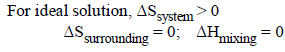*Multiple options can be correct
Test: MCQs (One or More Correct Option): Solutions | JEE Advanced - Question 17

Mixture(s) showing positive deviation from Raoult’s law at35 °C is (are) (JEE Adv. 2016)

Detailed Solution for Test: MCQs (One or More Correct Option): Solutions | JEE Advanced - Question 17

(A) H-bonding of methanol breaks when CCl4 is added so bonds become weaker, resulting positive deviation.
(B) Mixing of polar and non-polar liquids will produce a solution of weaker interaction, resulting positive deviation
(C) Ideal solution
(D) –ve deviation because stronger H-bond is formed.

## Chemistry 35 Years JEE Main & Advanced Past year Papers

122 docs|66 tests
 Use Code STAYHOME200 and get INR 200 additional OFF Use Coupon Code
Information about Test: MCQs (One or More Correct Option): Solutions | JEE Advanced Page
In this test you can find the Exam questions for Test: MCQs (One or More Correct Option): Solutions | JEE Advanced solved & explained in the simplest way possible. Besides giving Questions and answers for Test: MCQs (One or More Correct Option): Solutions | JEE Advanced, EduRev gives you an ample number of Online tests for practice

## Chemistry 35 Years JEE Main & Advanced Past year Papers

122 docs|66 tests